# Pre-Algebra : Irrational Numbers

## Example Questions

← Previous 1

### Example Question #1 : Irrational Numbers

Which of the following expressions is irrational?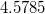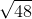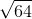Explanation:

An irrational number is defined as any number that cannot be expressed as a simple fraction or does not have terminating or repeating decimals. Of the answer choices given, the only number that cannot be expressed as a simple fraction or with repeating or terminating decimals is.

### Example Question #2 : Irrational Numbers

Which of the following is an irrational number?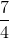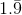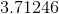Explanation:

An irrational number is any number that can not be expressed as a ratio of integers, i.e. a fraction. Therefore, the only irrational number listed is.

### Example Question #3 : Irrational Numbers

Which of these expressions is not irrational?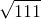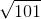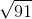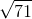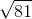Explanation:

The square root of an integer is either an irrational number or an integer. The latter is the case if and only if there is an integer which, when multiplied by itself, or squared, yields the number inside the symbol (the radicand) as the product. Of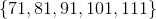, only 81 is the square of an integer (9).

### Example Question #1 : Irrational Numbers

Which of the following is closest to the value of the expression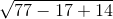?The expression is undefined in the real numbers.Explanation: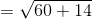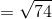Since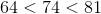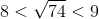.

We can determine which is closer by evaluating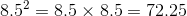.

Since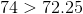, 9 is the closer integer, and it is the correct choice.

### Example Question #4 : Irrational Numbers

Which of the following represents an irrational number?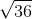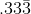All of the answers are irrational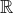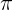Explanation:

Pi is the only irrational number listed. Irrational numbers are in the form of infinite non-repeating decimals.

### Example Question #1 : Understand The Difference Between Rational And Irrational Numbers: Ccss.Math.Content.8.Ns.A.1

Which of the following is not an irrational number?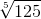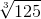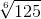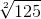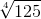Explanation:

A root of an integer is one of two things, an integer or an irrational number. By testing all five on a calculator, onlycomes up an exact integer - 5. This is the correct choice.

### Example Question #2 : Irrational Numbers

Which of the following numbers is an irrational number?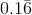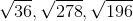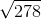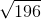Explanation:

An irrational number  is one that cannot be written as a fraction. All integers are rational numberes.

Repeating decimals are never irrational,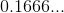can be eliminated because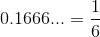.andare perfect squares making them both integers.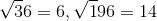Therefore, the only remaining answer is.

### Example Question #1 : Irrational Numbers

Which of the following is an irrational number?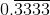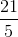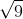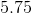Explanation:

A rational number can be expressed as a fraction of whole numbers, while an irrational number cannot.can be written as.is simply, which is a rational number.

The numbercan be rewritten as a fraction of whole numbers,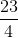, which makes it a rational number.is also a rational number because it is a ratio of whole numbers.

The number,, on the other hand, is irrational, since it has an irregular sequence of numbers (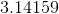...) that cannot be written as a fraction.

### Example Question #2 : Irrational Numbers

Which of the following is an irrational number?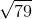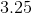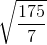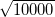Explanation:

An irrational number is any number that cannot be written as a fraction of whole numbers.  The number pi and square roots of non-perfect squares are examples of irrational numbers.can be written as the fraction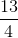.  The term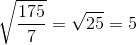is a whole number.  The square root of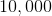is, also a rational number.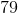, however, is not a perfect square, and its square root, therefore, is irrational.

### Example Question #1 : Irrational Numbers

Of the following, which is a rational number?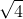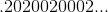Explanation:

A rational number is any number that can be expressed as a fraction/ratio, with both the numerator and denominator being integers. The one limitation to this definition is that the denominator cannot be equal to.

Using the above definition, we see,and(which is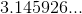) cannot be expressed as fractions. These are non-terminating numbers that are not repeating, meaning the decimal has no pattern and constantly changes. When a decimal is non-terminating and constantly changes, it cannot be expressed as a fraction.is the correct answer because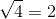, which can be expressed as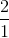, fullfilling our above defintion of a rational number.

← Previous 1

### All Pre-Algebra Resources